How to concatenate two strings in Javascript – The complete guide

In this tutorial, we’ll be looking at string concatenation: How you can concatenate two strings in Javascript, the different methods you can use for it, and some examples for each of those methods.

You can watch the video version of this tutorial here:

Create the base for programming

Let’s first create an index.html file and a script.js file and connect them. The title of the file is going to be “Javascript for beginners – String concatenation.

<!DOCTYPE html>
<!DOCTYPE html>
<html lang="en">
<meta charset="utf-8">
<meta name="viewport" content="width=device-width, initial-scale=1.0">
<title> Javascript for beginners – String concatenation </title>

<body>
<p id="para"></p>
<script src="script.js"></script>
</body>
</html>

So, that’s a HTML5 boilerplate template, with the page’s language set to English (en). We have a bunch of the usual meta tags as well.

In the body, we are connecting to the script file. We’ve also created a paragraph tag with the id “para”.

That’s all we have for now. We can now proceed with our examples.

So now, let’s move on to the script.js file, and start off with the concatenation. Now, string concatenation is basically joining two strings.

//String concatenation

You’ve got two different strings. You’re going to join them and merge them into one string. That’s what we’re trying to do here.

String concatenation “+” operator

In Javascript, you can do that in a multitude of ways, and one of them is by using the String concatenation operator, or the “+” operator.

Now, this “+” operator, if used with numbers, like this:

var a = 1 + 2;

that would just add these two numbers and give out 3 as the output.

But if the same operator is used with strings, it’ll concatenate them and display them together, side by side. Let me show you.

Let’s create two strings and assign two string values to them and create a 3rd variable to concatenate the two strings.

var a = "Hello";
var b = "World";
var c = a + b;
document.write(c);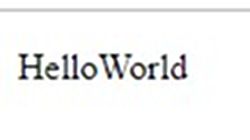Now, if we reverse the strings,

var c = b + a;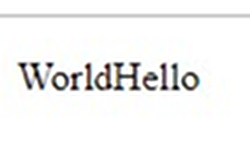This would be the output. So, the result of the concatenation of two strings is based on how the strings are arranged around the concatenation operator as well.

You can also add space between those two strings. Let’s create another string called d, and it’s going to hold the space we need.

var d = " ";

Now, we can concatenate all the 3 strings we’ve created so far.

var c = a + d + b;

This will be the output of the previous command: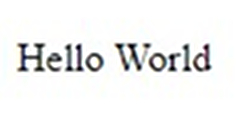This is how you can play around with your strings and the concatenation operator to get different results.

Direct concatenation

You don’t have to resort to assigning strings to variables, and then concatenating them. You can directly concatenate strings as well.

var c = "Hello" + " " + "World";

The above line of code will get you the same output.

Concatenating strings with numbers

You already know that using the “+” operator on numbers gives the addition of the two numbers.
But what happens when we use the same operator on a number and a string?

var c = 1 + "World";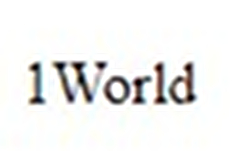It just concatenated the two strings. So, even when both the operators aren’t strings, even if one of them is a string, the “+” would automatically act as the concatenation operator.

So, what happens is, when the browser runs the program, it encounters the number 1 in the statement first. Then it’ll encounter the plus symbol, and so far, the browser would assume that it’d be asked to perform an addition operation.

But, as soon as it comes across the string “World”, it’ll completely disregard the idea of performing an addition operation, consider both operands as strings (even though 1 is a number) and perform a concatenation.

2 numbers and a string

On the other hand, how would the following line of code be interpreted by the browser?

var c = 1 + 2 + "World";

So, the output is: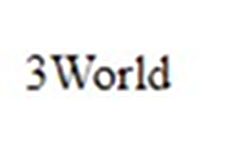Now what happens is, the browser comes across 1 and “+” and prepare for addition. It’ll come across 2 next and perform its first addition operation.

Now, the result of the first addition, 3, will be stored by the browser.

Next, when it comes across the string, it’ll stop performing addition and perform concatenation on the new result (3) and the string (“World”), which is why we end up with “3World” as the result.

String and 2 numbers

But what happens when we try to add a string, followed by two numbers?

var c = "World" + 1 + 2;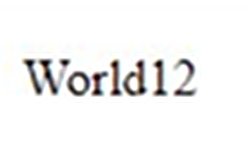The result would be a complete concatenation of all the operands involved, even though two of them were numbers.

Why is that?

When the browser encounters the string first, rather than a number, followed by the “+” symbol, it’ll immediately prepare for concatenation.

So, the result of the first concatenation would be “World1”.

Then, “World1”, which is also a string now, will be concatenated with 2, so we end up with “World12”.

The “+=” operator

Just like the “+” operator, the “+=” operator can be used for concatenation as well. If used with numbers, it’ll add the numbers and assign it to the variable at the left-hand side of the operator.

But when used with one or more strings, it’ll automatically transform into yet another string concatenation operator and concatenate the two operands, even if one of them is a number.

var a = "Hello";
var b = " World!";
a += b;
document.write(a);

So, let’s first create a variable a and assign it a string “Hello”. Then, let’s create another variable b, and assign it the string “ World!”. Notice how I’ve created a space before “World” and ended it with a “!”.

Then, let’s add a to b, and display a.

The expression a += b is just a short form for a = a + b.

So, that’ll basically be =>

a = a + " World!";

But the variable a has the value “Hello” right now, so the expression will now become,

a = "Hello" + " World!";

So now, a will hold the value “Hello World!”

You’ll get the following result when you run this program: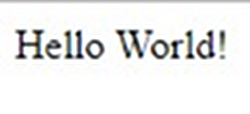What’s happening here is, the browser takes the value of a, which is a string, and concatenates it with the value of b, which is also a string, and assign the concatenated string to a.

Now, a will contain the concatenated string “Hello World!”. Do you see how we reduced one line of code by not having to create a 3rd variable c to assign the concatenated value to?

Concatenating with a single variable

Instead of creating two variables, you can do the above with just one variable as well.

var a = "Hello";
a += " World!";
document.write(a);

The above code will get you the same result as well.

In here, instead of a = a + b, we are performing the calculation for a = a + ” World!”.

The string.concat() pre-defined function

So far, we’ve been looking at rudimentary ways of concatenating strings by using mathematical operators.

But we do have a per-defined Javascript string function that does the exact same thing.

This is how the syntax for this function works:

var stringAns = string1.concat(string2);

So, the first string should be mentioned first, then place a period and the concat() function. Inside the parenthesis, mention the string you want to concatenate with the first string.

Make sure that you mention the strings in the correct order. The string mentioned before the concat() function call will always come first in the concatenation output.

Let’s look at an example:

var a = "Hello";
var b = "World";
var c = a.concat(b);
document.write(c);

As the code above specifies, we are trying to concatenate the string a with string b, and we are assigning the concatenated result to c. Since the variable a is mentioned first, the string stored inside this variable will be displayed first.

We get the following result when we run this code: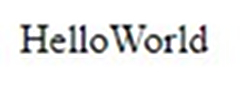Do you see how there is no space between the two words? What if I want a space, but don’t want to add the space in variable b? What if I want to add an exclamation point at the end as well?

Then we’ll need to concatenate more than 2 strings at a time with the same function because space and exclamation points will be considered strings as well.

This is how the syntax for that will look like:

Var stringAns = string1.concat(string2, string3, string4….);

Let’s look at the same with an example:

var a = "Hello";
var b = "World";
var c = a.concat(" ", b, "!");
document.write(c);

So now, I’ve added the strings ” ” (space) and “!” to my concatenation function, and I get the output “Hello World!”.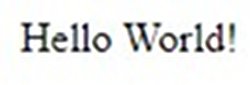Well, that’s it! We’ve looked at different ways of concatenating strings in Javascript. I personally prefer using the “+” operator, but you are free to choose whatever you want.